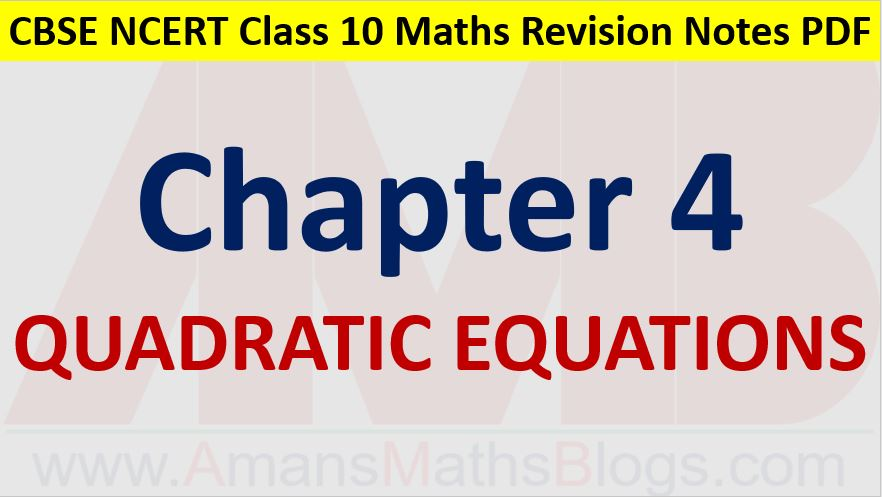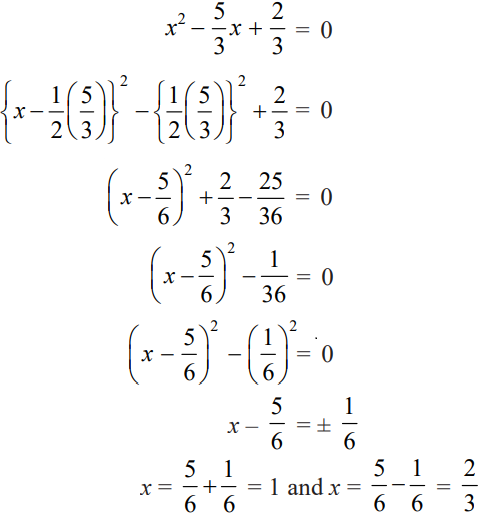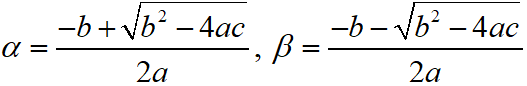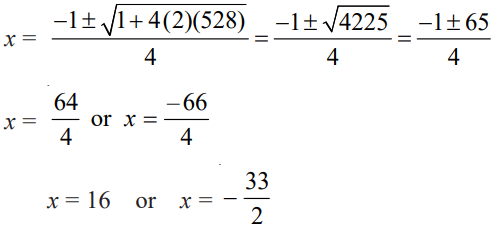Friday, October 22, 2021
Home > CBSE Class 10 > Quadratic Equations CBSE NCERT Notes Class 10 Maths PDF

# Quadratic Equations CBSE NCERT Notes Class 10 Maths PDFHi students, Welcome to AMBiPi (Amans Maths Blogs). In this article, you will get Quadratic Equations CBSE Notes Class 10 Maths Chapter 4 PDF. You can download this PDF and save it in your mobile device or laptop etc.

# Quadratic Equations CBSE Notes Class 10 Maths Chapter 4

The standard form of a quadratic polynomial is p(x) = ax2 + bx + c. Then, ax2 + bx + c = 0 is the general equation of quadratic equations, where a, b and c are real and a0.

Since the degree of a quadratic equation is two, then the quadratic equation is satisfied by exactly two roots which may be real of imaginary.

### Class 10 Maths Chapter 4 Examples 1:

Check whether the equation x3 – 4x2 – x + 1 = (x – 2)3 is quadratic or not.

x3 – 4x2 – x + 1 = (x – 2)3

⇒ x3 – 4x2 – x + 1 = x3 – 8 – 6x2 + 12x

⇒ – 4x2 – x + 1 = – 8 – 6x2 + 12x

⇒ 2x2 – 13x + 9 = 0

It is of the form ax2 + bx + c = 0. So, the given equation is a quadratic equation.

## Solution of Quadratic Equations : By Factorization

A real number x = α is a root of the quadratic equation ax2 + bx + c = 0, a ≠ 0 if α satisfies the quadratic equation it means, a α2 + bα + c = 0.

### Class 10 Maths Chapter 4 Examples 2:

Find the roots of the following quadratic equations by factorization: x2 – 3x – 10 = 0.

x2 – 3x – 10 = 0

⇒ x2 – 5x + 2x – 10 = 0

⇒ x(x – 5) + 2(x – 5) = 0

⇒ (x + 2)(x – 5) = 0

⇒ x = -2 or 5

## Solution of Quadratic Equations : By Method of Completing Square

### Class 10 Maths Chapter 4 Examples 2:

Find the roots of the following quadratic equations by method of completing the square: 3x2 – 5x + 2 = 0.

Given that 3x2 – 5x + 2 = 0The roots of the quadratic equation ax2 + bx + c = 0 areThus, the sum of the roots is α + β = -b/a and the product of the roots is αβ = c/a.

### Class 10 Maths Chapter 4 Examples 3:

Solve the quadratic equation 2x2 + x – 528 = 0 by using quadratic formula.

Given that a = 2, b = 1 and c = -528## Nature of Roots of Quadratic Equations

In a quadratic equation ax2 + bx + c = 0, the value D = b2 – 4ac is known as discriminant of the quadratic equation.

The nature of the roots of the quadratic equation depends on the discriminant.

Case I: When D > 0

In this case, the roots α and β of the quadratic equation are real and unequal.

Case II: When D = 0

In this case, the roots α and β of the quadratic equation are real and equal.

Case III: When D < 0

In this case, the roots α and β of the quadratic equation are imaginary and distinct.

### Class 10 Maths Chapter 4 Examples 4:

Find the nature of the roots of the quadratic equation 2x2 – 4x + 3 = 0.

Given that a = 2, b = -4 and c = 3.

Since the discriminant b2 – 4ac = (– 4)2 – (4 × 2 × 3) = 16 – 24 = – 8 < 0

Thus, So, the given equation has no real roots.

### Class 10 Maths Chapter 4 Examples 5:

Find the values of k for each of the quadratic equation 2x2 + kx + 3 = 0, so that they have two
equal roots.

Given that a = 2, b = k and c = 3.

Since the given equation has equal roots, then

D = b2 – 4ac = 0

⇒ k2 – (4 × 2 × 3) = 0

⇒ k2 = 24

⇒ k = 2√3.

Click below to get CBSE Class 10 Maths Chapter wise Revision Notes PDF.

Chapter NoChapter name
Chapter 1 :Real Numbers CBSE Class 10 Maths Revision Notes
Chapter 2 :Polynomials CBSE Class 10 Maths Revision Notes
Chapter 3 :Pair of Linear Equations in Two Variables CBSE Class 10 Maths Revision Notes
Chapter 4 :Quadratic Equations CBSE Class 10 Maths Revision Notes
Chapter 5 :Arithmetic Progression CBSE Class 10 Maths Revision Notes
Chapter 6 :Triangles CBSE Class 10 Maths Revision Notes
Chapter 7 :Coordinate Geometry CBSE Class 10 Maths Revision Notes
Chapter 8 :Introduction to Trigonometry CBSE Class 10 Maths Revision Notes
Chapter 9 :Some Applications of Trigonometry CBSE Class 10 Maths Revision Notes
Chapter 10 :Circles CBSE Class 10 Maths Revision Notes
Chapter 11 :Constructions CBSE Class 10 Maths Revision Notes
Chapter 12 :Area Related to Circles CBSE Class 10 Maths Revision Notes
Chapter 13 :Surface Areas & Volumes CBSE Class 10 Maths Revision Notes
Chapter 14 :Statistics CBSE Class 10 Maths Revision Notes
Chapter 15 :Probability CBSE Class 10 Maths Revision Notes
AMBiPi
error: Content is protected !!